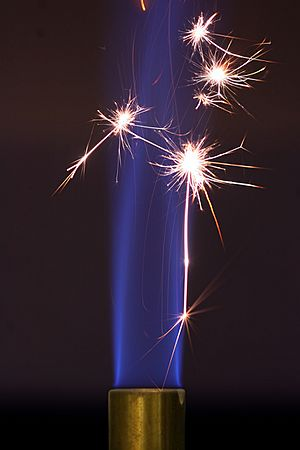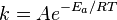# Activation energy facts for kids

Kids Encyclopedia FactsThe sparks created by striking steel against a piece of flint provide the activation energy to initiate combustion in this Bunsen burner. The blue flame sustains itself after the sparks stop because the continued combustion of the flame is now energetically favorable.

The activation energy of a chemical reaction is the minimum energy that is needed to make the reaction happen. It usually has the symbol Ea and it is measured in kilojoule per mole. It can be thought of as a barrier between the reagents and the products of a reaction. The activation energy is the difference in energy between the transition state and the starting reagents.

## Arrhenius equation

This equation is a way of calculating the activation energy. Its form is:$k = A e^{{-E_a}/{RT}}$

Here, k is the rate constant of the reaction (which can be measured using chemical kinetics), A is a frequency factor, R is the universal gas constant, T is the temperature. If we measure how fast the reaction goes at different temperatures, then we can make a graph and calculate the activation energy using this equation.

## Catalysis

When using a catalyst the activation energy is made smaller. This means that the reaction can go faster at the same temperature. The catalyst does not change the energy of the reagents or the products. Also, the catalyst does not change in the reaction. Therefore, it can be used again and again and only a small amount of a catalyst is needed.Activation energy Facts for Kids. Kiddle Encyclopedia.